ConicBundle
ConicBundle::AffineFunctionTransformation Class Reference

transform a function f(z) to fun_coeff*f(arg_offset+arg_trafo*y)+linear_cost*y+fun_offset (scales the value, substitutes argument z=c+Ay, and adds an affine term b'y+d) More...

#include <AffineFunctionTransformation.hxx>

Inheritance diagram for ConicBundle::AffineFunctionTransformation:## Public Member Functions

virtual int init (CH_Matrix_Classes::Real fun_coeff=1., CH_Matrix_Classes::Real fun_offset=0., CH_Matrix_Classes::Matrix *linear_cost=0, CH_Matrix_Classes::Matrix *arg_offset=0, CH_Matrix_Classes::Sparsemat *arg_trafo=0, bool model_calls_delete=true)
sets the parameters of the transformation. The ownership of objects pointed to is passed to *this (they will be deleted here). If *this is entered into an AFTModel, model_calls_delete==true tells the AFTModel to delete this AffineFunctionTransformation at the end.

AffineFunctionTransformation (CH_Matrix_Classes::Real in_fun_coeff=1., CH_Matrix_Classes::Real in_fun_offset=0., CH_Matrix_Classes::Matrix *in_linear_cost=0, CH_Matrix_Classes::Matrix *in_arg_offset=0, CH_Matrix_Classes::Sparsemat *in_arg_trafo=0, bool in_model_calls_delete=true, CBout *cbo=0, int incr=-1)
calls init()

virtual ~AffineFunctionTransformation ()
deletes linear_cost, arg_offset and arg_trafo

bool get_model_calls_delete ()
retruns true if the model has to delete this

void set_model_calls_delete (bool mcd)
set to true if the model has to delete this, to false if it is destructed elsewhere

bool argument_changes () const
returns true if not the identity

bool sparse_argument_changes () const
returns true if arg_trafo influences at most two thirds of the entries of local_argument

bool scaled_index_subset (const CH_Matrix_Classes::Indexmatrix *col_ind, const CH_Matrix_Classes::Indexmatrix *row_ind) const
returns true if the transformation maps each index (in col_ind if !=0) onto at most one index and vice versa (out of row_ind if !=0)

bool scaled_index (CH_Matrix_Classes::Integer &mapped_index, CH_Matrix_Classes::Real &coeff, CH_Matrix_Classes::Integer index) const
returns false if index is mapped to more than one index, otherwise true with mapped_index==-1 if mapped to zero, else >=0 and coeff gives the coefficient

CH_Matrix_Classes::Real get_fun_coeff () const
returns the factor for the function

CH_Matrix_Classes::Realset_fun_coeff ()
allows to set the factor for the function

CH_Matrix_Classes::Real get_fun_offset () const
returns the constant offset for the funciton

CH_Matrix_Classes::Realset_fun_offset ()
allows to set the constant offset for the funciton

const CH_Matrix_Classes::Matrixget_linear_cost () const
returns the pointer to the linear term added to the funciton

const CH_Matrix_Classes::Matrixget_arg_offset () const
returns the pointer to the constant offset added to the argument (not neeeded in the code)

const CH_Matrix_Classes::Sparsematget_arg_trafo () const
returns the pointer to the linear transformation of the argument (not neeeded in the code)

CH_Matrix_Classes::Real get_linear_cost (CH_Matrix_Classes::Integer i) const
returns the value of the linear cost coefficient for i>=0 and for i==-1 the constant offset

const MinorantPointerget_constant_minorant () const
return the constant linear minorant corresponding to fun_offset+<*linear_cost,.>

CH_Matrix_Classes::Integer from_dim () const
returns the dimension of the input argument or -1 if it is unknown

CH_Matrix_Classes::Integer to_dim () const
returns the dimension of the output argument or -1 if it is unknown

int copy_traforows (CH_Matrix_Classes::Matrix &copy_to, const CH_Matrix_Classes::Matrix &copy_from) const
only copies the elements that are effected by the image of arg_trafo

const CH_Matrix_Classes::Matrixtransform_argument (CH_Matrix_Classes::Matrix &transformed_y, CH_Matrix_Classes::Real &transformed_offset, const CH_Matrix_Classes::Matrix &input_y) const
computes transformed_offset and, if this is not the identity,transformed_y and returns either input_y (id) or transformed_y More...

const CH_Matrix_Classes::Matrixmodified_transform_argument (CH_Matrix_Classes::Matrix &transformed_y, const CH_Matrix_Classes::Matrix &input_y, const AFTModification *aftmdf, const GroundsetModification &gsmdf) const
given the modification aftmdf or if 0, gsmdf, compute the transformed argument that would arise after this modification as in transform_argument() More...

CH_Matrix_Classes::Real objective_value (CH_Matrix_Classes::Real offset, CH_Matrix_Classes::Real function_value) const
if offset is the value computed in transform_argument and function_value results form an evaluation in the respective point, the routine returns the objective value obtained by the affine transformation

int transform_minorant (MinorantPointer &out_minorant, const MinorantPointer &in_minorant, CH_Matrix_Classes::Real alpha=1., bool add_trafo_minorant=false, const CH_Matrix_Classes::Indexmatrix *provided_row_indices=0, const CH_Matrix_Classes::Indexmatrix *needed_column_indices=0) const
transforms the in linear minorant (scaled by alpha) and initializes or adds it to the out linear minorant More...

int transform_minorants (MinorantBundle &out_minorants, const MinorantBundle &in_minorants, CH_Matrix_Classes::Real alpha=1.) const
transforms several linear minorants (scaled by alpha) and initializes or adds them to the out linear minorants More...

int qp_cost_indices (CH_Matrix_Classes::Indexmatrix &provide_row_indices, const CH_Matrix_Classes::Indexmatrix *needed_col_indices) const
if the algorithm only requires the indices given in needed_col_indices (NULL means all indices) then it suffices to supply the provide_row_indices as indices in transform_minorant (a 0x0 return matrix again means all indices, while a 0x1 matrix means no indices). Any input or output indices must be in strictly increasing order.

int scaling_indices (CH_Matrix_Classes::Indexmatrix &row_indices, const CH_Matrix_Classes::Indexmatrix &col_indices) const
if AFTModel::add_diagonal_scaling() is called with indices specified by col_indices, then AFTmodel has to provide a diagonal scaling matrix of dimension to_dim() as in_diagscale in add_diagonal_scaling() with the entries in the output vector row_indices computed correctly.

int add_diagonal_scaling (CH_Matrix_Classes::Matrix &diagscale, const CH_Matrix_Classes::Indexmatrix *indices, CH_Matrix_Classes::Real alpha, const CH_Matrix_Classes::Matrix &in_diagscale) const
transform the scaling matrix in_diagscale of the untransformed function model and add it as described in BundleMethod::add_diagonal_scaling. More...

const GroundsetModificationanalyze_modification (bool &minorant_trafo_differs, const AFTModification *aftmdf, const GroundsetModification &gsmdf) const
returns information on the changes in the ground set of the transformed arguments and checks whether after the modification the nonzero image of the transformed minorants will not differ from before; returns NULL on errors. More...

int apply_modification (const AFTModification *aftmdf, const GroundsetModification &gsmdf)
if arg_trafo==NULL (act as identity) and if aftmdf!=NULL adds any explicit matrix parts or has modifications not consistent with maintaining the identity, arg_trafo is first set to an explicit identity before executing the modification. If arg_trafo==NULL and aftmdf==NULL, the transformations of gsmdf are applied to linear_cost and arg_offset.

virtual std::ostream & output_aft_data (std::ostream &out) const
for testing purposes this outputs the data in mfile readable formPublic Member Functions inherited from ConicBundle::CBout
virtual void set_out (std::ostream *out=0, int print_level=1)
Specifies the output level (out==NULL: no output at all, out!=NULL and level=0: errors and warnings, level>0 increasingly detailed information) More...

virtual void set_cbout (const CBout *cb, int incr=-1)
Specifies the output level relative to the given CBout class. More...

void clear_cbout ()
reset to default settings (out=0,print_level=1)

CBout (const CBout *cb=0, int incr=-1)
calls set_cbout

CBout (std::ostream *outp, int pl=1)
initialize correspondingly

CBout (const CBout &cb, int incr=0)
copy constructor

virtual bool cb_out (int pl=-1) const
Returns true if out!=0 and (pl<print_level), pl<0 should be used for WARNINGS and ERRORS only, pl==0 for usual output.

std::ostream & get_out () const
If cb_out() returned true, this returns the output stream, but it will abort if called with out==0.

std::ostream * get_out_ptr () const
returns the pointer to the output stream

int get_print_level () const
returns the print_level

virtual int mfile_data (std::ostream &out) const
writes problem data to the given outstream

## Private Attributes

CH_Matrix_Classes::Real fun_coeff
function value is multiplied by this

CH_Matrix_Classes::Real fun_offset
constant added in the end

CH_Matrix_Classes::Matrixlinear_cost
NULL means no linear cost.

CH_Matrix_Classes::Matrixarg_offset
NULL means no offset.

CH_Matrix_Classes::Sparsematarg_trafo
NULL means identity (!!!)

MinorantPointer constant_minorant
corresponds to fun_offset + <*linear_cost,.>

GroundsetModification local_gsmdf
this describes the effect of the last modification on the image space (if there was one at all)

bool model_calls_delete
tells the model whether it should delete this at the end of its use or just leave it alone (i.e., this AFT is then owned by someone else)

## Detailed Description

transform a function f(z) to fun_coeff*f(arg_offset+arg_trafo*y)+linear_cost*y+fun_offset (scales the value, substitutes argument z=c+Ay, and adds an affine term b'y+d)

The AffineFunctionTransformation (AFT for short) allows to use an implemented function oracle for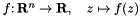as a function oracle of the function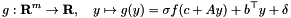where

•(Real fun_coeff, default value 1) scales the function value (0 is allowed to cancel the function for cases where removing it might cause difficulties)
•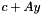specifies an affine transformation of the argument with
•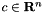(Matrix pointed to by arg_offset, NULL pointer represents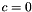)
•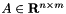(Sparsemat pointed to by arg_trafo, NULL pointer represents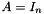, so indeed the IDENTITY and NOT the zero matrix)
•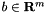(Matrix pointed to by linear_cost, NULL pointer represents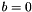) adds a linear cost term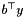•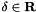(Real fun_offset, default value=0) adds a constant offset

If (some of) the pointers to matrices are specified, ownership of the matrix objects pointed to will be taken over by the AFT and the objects will be deleted by this class at the end of their use.

Note, if arg_offset and arg_trafo are both zero, then the argument does not change at all. If all three matrix pointers are zero, there is no information on the dimension of the transformation, so it will be determined by the arguments supplied.

Using value 0. for fun_coeff is allowed and removes the influence of the function completely, leaving only the affine function in y specified by fun_offset and linear_cost.

Internally, the SumBlockModel realization of the AFT is achieved by AFTModel.

## Member Function Documentation

 int ConicBundle::AffineFunctionTransformation::add_diagonal_scaling ( CH_Matrix_Classes::Matrix & diagscale, const CH_Matrix_Classes::Indexmatrix * indices, CH_Matrix_Classes::Real alpha, const CH_Matrix_Classes::Matrix & in_diagscale ) const

transform the scaling matrix in_diagscale of the untransformed function model and add it as described in BundleMethod::add_diagonal_scaling.

If indices are given, they must be sorted in striclty increasing order.

Referenced by objective_value().

## ◆ analyze_modification()

 const GroundsetModification* ConicBundle::AffineFunctionTransformation::analyze_modification ( bool & minorant_trafo_differs, const AFTModification * aftmdf, const GroundsetModification & gsmdf ) const

returns information on the changes in the ground set of the transformed arguments and checks whether after the modification the nonzero image of the transformed minorants will not differ from before; returns NULL on errors.

If arg_trafo==NULL (act as identity) and if aftmdf!=NULL adds any explicit matrix parts or has modifications not consistent with maintaining the identity, arg_trafo is virtually first set to an explicit identity before executing the modification. If arg_trafo==NULL and aftmdf==NULL, the transformations of gsmdf are applied to linear_cost and arg_offset.

If the input gsmdf and the AFT modifications in aftmdf result in a different GroundsetModification of the transformed space, this is computed into the member local_gsmdf and the returned pointer points to this local_gsmdf. If all transformations are passed on exactly as in gsmdf (because the trafo still acts as the identity), the returned pointer points to gsmdf.

If the changes affect the transformation at all in its effect on the nonzero image of transformed minorants, minorant_trafo_differs will be set to true otherwise to false.

Referenced by objective_value().

## ◆ modified_transform_argument()

 const CH_Matrix_Classes::Matrix& ConicBundle::AffineFunctionTransformation::modified_transform_argument ( CH_Matrix_Classes::Matrix & transformed_y, const CH_Matrix_Classes::Matrix & input_y, const AFTModification * aftmdf, const GroundsetModification & gsmdf ) const

given the modification aftmdf or if 0, gsmdf, compute the transformed argument that would arise after this modification as in transform_argument()

On input transformed_y is supposed to be of dimension zero. The matrix returned is transformed_y unless the modification preserves the identity transformation. In this case, the returned matrix is input_y.

Referenced by to_dim().

## ◆ transform_argument()

 const CH_Matrix_Classes::Matrix& ConicBundle::AffineFunctionTransformation::transform_argument ( CH_Matrix_Classes::Matrix & transformed_y, CH_Matrix_Classes::Real & transformed_offset, const CH_Matrix_Classes::Matrix & input_y ) const

computes transformed_offset and, if this is not the identity,transformed_y and returns either input_y (id) or transformed_y

On input transformed_y is supposed to be of dimension zero, unless it was already the result of a previous transformation for exactly this AFT with this data (no intermediate modifications). This is important, because if transformed_y has the correct size, only the data changed by the transformation is overwritten and the constant parts are assumed to be already initialized. On output it is again of dimension 0 if the transformation is the identity.

The transformed_offset = fun_offset+ip(linear_cost,input_y) is the value needed in objective_value() together with the result of the evaluation of the function for transformed_y in order to compute the transformed objective value.

Referenced by to_dim().

## ◆ transform_minorant()

 int ConicBundle::AffineFunctionTransformation::transform_minorant ( MinorantPointer & out_minorant, const MinorantPointer & in_minorant, CH_Matrix_Classes::Real alpha = 1., bool add_trafo_minorant = false, const CH_Matrix_Classes::Indexmatrix * provided_row_indices = 0, const CH_Matrix_Classes::Indexmatrix * needed_column_indices = 0 ) const

transforms the in linear minorant (scaled by alpha) and initializes or adds it to the out linear minorant

if out_minorant.empty()==true, the out_minorant is initialized, otherwise the information is added.

the constant_minorant of the transformation is only added if add_trafo_minorant is set to true.

If given, provided_row_indices and needed_column_indices must be as specified in qp_cost_indices, i.e. of in_minorant only the provided_row_indices (all if NULL) will be used to compute only the needed_column_indices (as rows, all if NULL) of out_minorant.

Referenced by objective_value().

## ◆ transform_minorants()

 int ConicBundle::AffineFunctionTransformation::transform_minorants ( MinorantBundle & out_minorants, const MinorantBundle & in_minorants, CH_Matrix_Classes::Real alpha = 1. ) const

transforms several linear minorants (scaled by alpha) and initializes or adds them to the out linear minorants

for out_minorant[i].empty()==true the out_minorant is initialized, otherwise the information is added.

Referenced by objective_value().

The documentation for this class was generated from the following file: